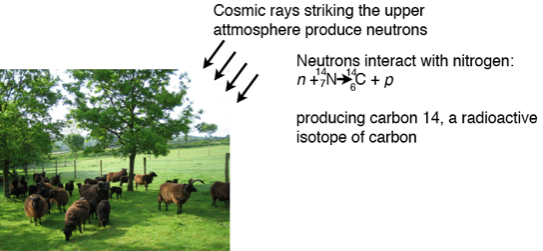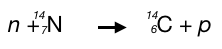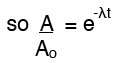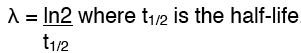Radioactive carbon 14 is the key

Very fast moving particles emitted by the Sun (cosmic rays) hit the atoms in the upper atmosphere and changes some of them.Neutrons from the cosmic rays interact with nitrogen atomsThese carbon atoms (carbon 14) are unstable, they are radioactive but with a long half life. The carbon will combine with oxygen in the atmosphere to form carbon dioxide.

Carbon dioxide is absorbed by plants and therefore some of the carbon dioxide absorbed contains carbon 14 and is radioactive. These plant and either die and decay or they may be eaten by animal. Either way, no more carbon dioxide is absorbed. The radioactive carbon will gradually decay but it has a very long half life of 5740 years.

If we then have an ancient sample of organic material we can measure the radioactivity due to carbon 14 and compare that to the amount we would expect it to have when it was alive. From this we can calculate the age.

Calculation example

For example:

A sample of timber from an archeological dig showed the presence of 64% of the carbon 14 atoms that would have been expected in fresh wood. Estimate the age of the sample, given that the half life of carbon 14 is 5730 years

The half life of carbon 14 is 5730 years.

where Ao is the original activity and A is the activity now, e is the constant base of natural logarithm and λ the decay constant for carbon 14.

Since the amount of radioactivity now has been measured at 64% of the original then Ao/A = 0.64:also equals 0.64, so rearranging this:

-λt = ln0.64 and t = -ln0.64/λ

NowThat gives λ = 0.693/5730 = 0.000121yrs-1

therefore t = -ln0.64/0.000121 = 3688 years

There are more useful pages about different aspects and uses of radioactivity here: